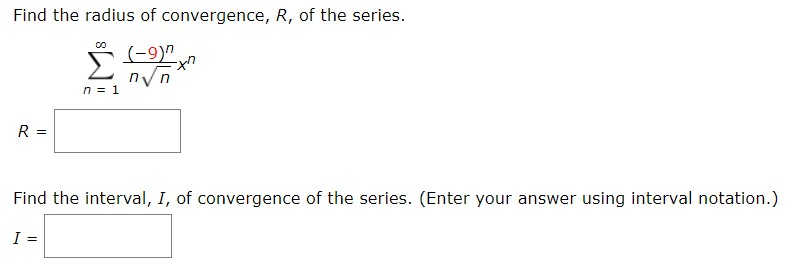# Find the radius of convergence, R, of the series.(-9)n 1R =Find the interval, I, of convergence of the series. (Enter your answer using interval notation.)I =

Question
1 viewshelp_outlineImage TranscriptioncloseFind the radius of convergence, R, of the series. (-9) n 1 R = Find the interval, I, of convergence of the series. (Enter your answer using interval notation.) I = fullscreen
check_circle

Step 1

If the interval of the convergence is (a,b) then radius of convergence will be (b-a)/2

According to ratio test for interval of convergence, the condition that should be satisfying is,

Step 2

Find an in simplified form.

Step 3

Find the ratio of an+1 and a...

### Want to see the full answer?

See Solution

#### Want to see this answer and more?

Solutions are written by subject experts who are available 24/7. Questions are typically answered within 1 hour.*

See Solution
*Response times may vary by subject and question.
Tagged in

### Math